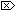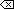The Open Group Base Specifications Issue 6
IEEE Std 1003.1-2001

#### NAME

strtod, strtof, strtold - convert a string to a double-precision number

#### SYNOPSIS

```#include <stdlib.h> double strtod(const char *restrict nptr, char **restrict endptr); float strtof(const char *restrict nptr, char **restrict endptr); long double strtold(const char *restrict nptr, char **restrict endptr); ```

#### DESCRIPTION

[CX]The functionality described on this reference page is aligned with the ISO C standard. Any conflict between the requirements described here and the ISO C standard is unintentional. This volume of IEEE Std 1003.1-2001 defers to the ISO C standard.These functions shall convert the initial portion of the string pointed to by nptr to double, float, and long double representation, respectively. First, they decompose the input string into three parts:

1. An initial, possibly empty, sequence of white-space characters (as specified by isspace())

2. A subject sequence interpreted as a floating-point constant or representing infinity or NaN

3. A final string of one or more unrecognized characters, including the terminating null byte of the input string

Then they shall attempt to convert the subject sequence to a floating-point number, and return the result.

The expected form of the subject sequence is an optional plus or minus sign, then one of the following:

• A non-empty sequence of decimal digits optionally containing a radix character, then an optional exponent part

• A 0x or 0X, then a non-empty sequence of hexadecimal digits optionally containing a radix character, then an optional binary exponent part

• One of INF or INFINITY, ignoring case

• One of NAN or NAN(n-char-sequenceopt), ignoring case in the NAN part, where:

```n-char-sequence:
digit
nondigit
n-char-sequence digit
n-char-sequence nondigit

```

The subject sequence is defined as the longest initial subsequence of the input string, starting with the first non-white-space character, that is of the expected form. The subject sequence contains no characters if the input string is not of the expected form.

If the subject sequence has the expected form for a floating-point number, the sequence of characters starting with the first digit or the decimal-point character (whichever occurs first) shall be interpreted as a floating constant of the C language, except that the radix character shall be used in place of a period, and that if neither an exponent part nor a radix character appears in a decimal floating-point number, or if a binary exponent part does not appear in a hexadecimal floating-point number, an exponent part of the appropriate type with value zero is assumed to follow the last digit in the string. If the subject sequence begins with a minus sign, the sequence shall be interpreted as negated. A character sequence INF or INFINITY shall be interpreted as an infinity, if representable in the return type, else as if it were a floating constant that is too large for the range of the return type. A character sequence NAN or NAN(n-char-sequenceopt) shall be interpreted as a quiet NaN, if supported in the return type, else as if it were a subject sequence part that does not have the expected form; the meaning of the n-char sequences is implementation-defined. A pointer to the final string is stored in the object pointed to by endptr, provided that endptr is not a null pointer.

If the subject sequence has the hexadecimal form and FLT_RADIX is a power of 2, the value resulting from the conversion is correctly rounded.

[CX]The radix character is defined in the program's locale (category LC_NUMERIC ). In the POSIX locale, or in a locale where the radix character is not defined, the radix character shall default to a period ( '.' ).In other than the C [CX]or POSIXlocales, other implementation-defined subject sequences may be accepted.

If the subject sequence is empty or does not have the expected form, no conversion shall be performed; the value of str is stored in the object pointed to by endptr, provided that endptr is not a null pointer.

[CX]The strtod() function shall not change the setting of errno if successful.

Since 0 is returned on error and is also a valid return on success, an application wishing to check for error situations should set errno to 0, then call strtod(), strtof(), or strtold(), then check errno.#### RETURN VALUE

Upon successful completion, these functions shall return the converted value. If no conversion could be performed, 0 shall be returned, and errno may be set to [EINVAL].

If the correct value is outside the range of representable values, HUGE_VAL, HUGE_VALF, or HUGE_VALL shall be returned (according to the sign of the value), and errno shall be set to [ERANGE].

If the correct value would cause an underflow, a value whose magnitude is no greater than the smallest normalized positive number in the return type shall be returned and errno set to [ERANGE].

#### ERRORS

These functions shall fail if:

[ERANGE]
The value to be returned would cause overflow [CX]or underflow.These functions may fail if:

[EINVAL]
[CX]No conversion could be performed.The following sections are informative.

None.

#### APPLICATION USAGE

If the subject sequence has the hexadecimal form and FLT_RADIX is not a power of 2, and the result is not exactly representable, the result should be one of the two numbers in the appropriate internal format that are adjacent to the hexadecimal floating source value, with the extra stipulation that the error should have a correct sign for the current rounding direction.

If the subject sequence has the decimal form and at most DECIMAL_DIG (defined in <float.h>) significant digits, the result should be correctly rounded. If the subject sequence D has the decimal form and more than DECIMAL_DIG significant digits, consider the two bounding, adjacent decimal strings L and U, both having DECIMAL_DIG significant digits, such that the values of L, D, and U satisfy L <= D <= U. The result should be one of the (equal or adjacent) values that would be obtained by correctly rounding L and U according to the current rounding direction, with the extra stipulation that the error with respect to D should have a correct sign for the current rounding direction.

The changes to strtod() introduced by the ISO/IEC 9899:1999 standard can alter the behavior of well-formed applications complying with the ISO/IEC 9899:1990 standard and thus earlier versions of the base documents. One such example would be:

```int
what_kind_of_number (char *s)
{
char *endp;
double d;
long l;

d = strtod(s, &endp);
if (s != endp && *endp == `\0')
printf("It's a float with value %g\n", d);
else
{
l = strtol(s, &endp, 0);
if (s != endp && *endp == `\0')
printf("It's an integer with value %ld\n", 1);
else
return 1;
}
return 0;
}

```

If the function is called with:

```what_kind_of_number ("0x10")

```

an ISO/IEC 9899:1990 standard-compliant library will result in the function printing:

```It's an integer with value 16

```

With the ISO/IEC 9899:1999 standard, the result is:

```It's a float with value 16

```

The change in behavior is due to the inclusion of floating-point numbers in hexadecimal notation without requiring that either a decimal point or the binary exponent be present.

None.

#### FUTURE DIRECTIONS

None.

isspace() , localeconv() , scanf() , setlocale() , strtol() , the Base Definitions volume of IEEE Std 1003.1-2001, Chapter 7, Locale, <float.h>, <stdlib.h>

#### CHANGE HISTORY

First released in Issue 1. Derived from Issue 1 of the SVID.

#### Issue 5

The DESCRIPTION is updated to indicate that errno is not changed if the function is successful.

#### Issue 6

Extensions beyond the ISO C standard are marked.

The following new requirements on POSIX implementations derive from alignment with the Single UNIX Specification:

• In the RETURN VALUE and ERRORS sections, the [EINVAL] optional error condition is added if no conversion could be performed.

The following changes are made for alignment with the ISO/IEC 9899:1999 standard:

• The strtod() function is updated.

• The strtof() and strtold() functions are added.

• The DESCRIPTION is extensively revised.

ISO/IEC 9899:1999 standard, Technical Corrigendum No. 1 is incorporated.

End of informative text.

UNIX ® is a registered Trademark of The Open Group.
POSIX ® is a registered Trademark of The IEEE.
[ Main Index | XBD | XCU | XSH | XRAT ]